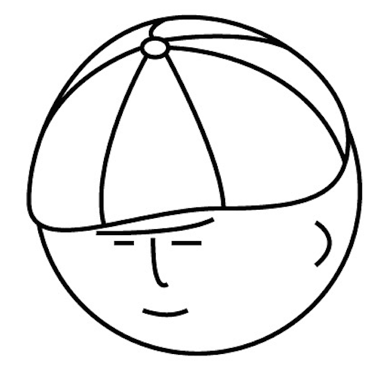# マツシタのお勉強メモ

#### マツシタのお勉強

##### welcome to my engineering blog
###### Hacker Rank# Problem

Given a sorted array of n integers that has been rotated an unknown number of times, write code to find an element int the array. You may assume that the array was originally sorted in increasing order.

# Solution

This problem can be solved by applying binary search. If the middle element is larger than the left element (arr[left] < arr[mid]), the left side is normally ordered. If the middle element is smaller than the left element (arr[left] > arr[mid]), the right side is normally ordered. If the left side is ordered and the x that we want to know the index is contained in the left side, we search the left side.

This solution takes O(log N) time.